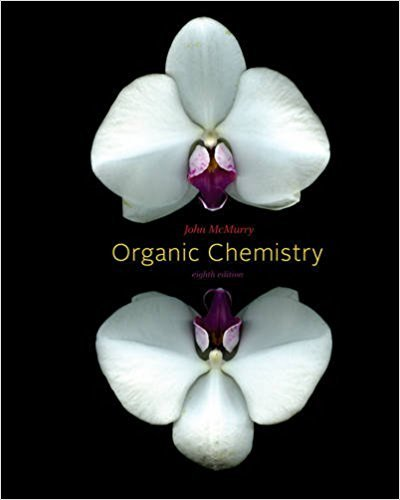×
×

# Solutions for Chapter 14.5: Characteristics of the DielsAlder Reaction## Full solutions for Organic Chemistry | 8th Edition

ISBN: 9780840054449Solutions for Chapter 14.5: Characteristics of the DielsAlder Reaction

Solutions for Chapter 14.5
4 5 0 373 Reviews
19
2
##### ISBN: 9780840054449

Organic Chemistry was written by and is associated to the ISBN: 9780840054449. This textbook survival guide was created for the textbook: Organic Chemistry, edition: 8. This expansive textbook survival guide covers the following chapters and their solutions. Since 4 problems in chapter 14.5: Characteristics of the DielsAlder Reaction have been answered, more than 46186 students have viewed full step-by-step solutions from this chapter. Chapter 14.5: Characteristics of the DielsAlder Reaction includes 4 full step-by-step solutions.

Key Business Terms and definitions covered in this textbook
• central bank

an institution designed to oversee the banking system and regulate the quantity of money in the economy

• circular-flow diagram

a visual model of the economy that shows how dollars flow through markets among households and firms

• competitive market

a market with many buyers and sellers trading identical products so that each buyer and seller is a price taker

• complements

two goods for which an increase in the price of one leads to a decrease in the demand for the other

• discount rate

the interest rate on the loans that the Fed makes to banks

• economics

the study of how society manages its scarce resources economies of scale the property whereby long-run average total cost falls as the quantity of output increases

• equilibrium

a situation in which the market price has reached the level at which quantity supplied equals quantity demanded

• imports

goods produced abroad and sold domestically

• income elasticity of demand

a measure of how much the quantity demanded of a good responds to a change in consumers’ income, computed as the percentage change in quantity demanded divided by the percentage change in income

• natural level of output

the production of goods and services that an economy achieves in the long run when unemployment is at its normal rate

• normal good

a good for which, other things being equal, an increase in

• perfect complements

two goods with right-angle indifference curves

• political economy

the study of government using the analytic methods of economics

• price elasticity of demand

a measure of how much the quantity demanded of a good responds to a change in the price of that good, computed as the percentage change in quantity demanded divided by the percentage change in price

• producer price index

a measure of the cost of a basket of goods and services bought by firms

• real GDP

the production of goods and services valued at constant prices

• shortage

a situation in which quantity demanded is greater than quantity supplied

• tax incidence

the manner in which the burden of a tax is shared among participants in a market

• total revenue (for a firm)

the amount a firm receives for the sale of its output

• Tragedy of the Commons

a parable that illustrates why common resources are used more than is desirable from the standpoint of society as a whole

×